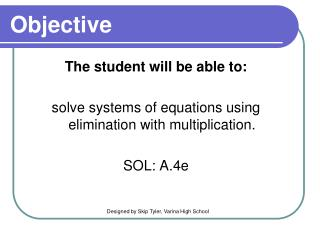DownloadDownload PresentationObjective

# Objective

Download Presentation## Objective

- - - - - - - - - - - - - - - - - - - - - - - - - - - E N D - - - - - - - - - - - - - - - - - - - - - - - - - - -
##### Presentation Transcript

1. Objective The student will be able to: solve systems of equations using elimination with multiplication. SOL: A.4e Designed by Skip Tyler, Varina High School

2. Solving Systems of Equations • So far, we have solved systems using graphing, substitution, and elimination. These notes go one step further and show how to use ELIMINATION with multiplication. • What happens when the coefficients are not the same? • We multiply the equations to make them the same! You’ll see…

3. Solving a system of equations by elimination using multiplication. Standard Form: Ax + By = C Step 1: Put the equations in Standard Form. Step 2: Determine which variable to eliminate. Look for variables that have the same coefficient. Step 3: Multiply the equations and solve. Solve for the variable. Step 4: Plug back in to find the other variable. Substitute the value of the variable into the equation. Step 5: Check your solution. Substitute your ordered pair into BOTH equations.

4. 1) Solve the system using elimination. 2x + 2y = 6 3x – y = 5 Step 1: Put the equations in Standard Form. They already are! None of the coefficients are the same! Find the least common multiple of each variable. LCM = 6x, LCM = 2y Which is easier to obtain? 2y(you only have to multiplythe bottom equation by 2) Step 2: Determine which variable to eliminate.

5. 1) Solve the system using elimination. 2x + 2y = 6 3x – y = 5 Multiply the bottom equation by 2 2x + 2y = 6 (2)(3x – y = 5) 8x = 16 x = 2 2x + 2y = 6 (+) 6x – 2y = 10 Step 3: Multiply the equations and solve. 2(2) + 2y = 6 4 + 2y = 6 2y = 2 y = 1 Step 4: Plug back in to find the other variable.

6. 1) Solve the system using elimination. 2x + 2y = 6 3x – y = 5 (2, 1) 2(2) + 2(1) = 6 3(2) - (1) = 5 Step 5: Check your solution. Solving with multiplication adds one more step to the elimination process.

7. 2) Solve the system using elimination. x + 4y = 7 4x – 3y = 9 Step 1: Put the equations in Standard Form. They already are! Find the least common multiple of each variable. LCM = 4x, LCM = 12y Which is easier to obtain? 4x(you only have to multiplythe top equation by -4 to make them inverses) Step 2: Determine which variable to eliminate.

8. 2) Solve the system using elimination. x + 4y = 7 4x – 3y = 9 Multiply the top equation by -4 (-4)(x + 4y = 7) 4x – 3y = 9) y = 1 -4x – 16y = -28 (+) 4x – 3y = 9 Step 3: Multiply the equations and solve. -19y = -19 x + 4(1) = 7 x + 4 = 7 x = 3 Step 4: Plug back in to find the other variable.

9. 2) Solve the system using elimination. x + 4y = 7 4x – 3y = 9 (3, 1) (3) + 4(1) = 7 4(3) - 3(1) = 9 Step 5: Check your solution.

10. What is the first step when solving with elimination? • Add or subtract the equations. • Multiply the equations. • Plug numbers into the equation. • Solve for a variable. • Check your answer. • Determine which variable to eliminate. • Put the equations in standard form.

11. Which variable is easier to eliminate? • x • y • 6 • 4 3x + y = 4 4x + 4y = 6

12. 3) Solve the system using elimination. 3x + 4y = -1 4x – 3y = 7 Step 1: Put the equations in Standard Form. They already are! Find the least common multiple of each variable. LCM = 12x, LCM = 12y Which is easier to obtain? Either! I’ll pick y because the signs are already opposite. Step 2: Determine which variable to eliminate.

13. 3) Solve the system using elimination. 3x + 4y = -1 4x – 3y = 7 Multiply both equations (3)(3x + 4y = -1) (4)(4x – 3y = 7) x = 1 9x + 12y = -3 (+) 16x – 12y = 28 Step 3: Multiply the equations and solve. 25x = 25 3(1) + 4y = -1 3 + 4y = -1 4y = -4 y = -1 Step 4: Plug back in to find the other variable.

14. 3) Solve the system using elimination. 3x + 4y = -1 4x – 3y = 7 (1, -1) 3(1) + 4(-1) = -1 4(1) - 3(-1) = 7 Step 5: Check your solution.

15. What is the best number to multiply the top equation by to eliminate the x’s? • -4 • -2 • 2 • 4 3x + y = 4 6x + 4y = 6

16. Solve using elimination. • (2, 1) • (1, -2) • (5, 3) • (-1, -1) 2x – 3y = 1 x + 2y = -3

17. Find two numbers whose sum is 18 and whose difference 22. • 14 and 4 • 20 and -2 • 24 and -6 • 30 and 8